# Rayleigh-Faber-Krahn inequality

An inequality concerning the lowest eigenvalue of the Laplace operator, with Dirichlet boundary condition, on a bounded domain in().

Letbe the Dirichlet eigenvalues of the Laplacian in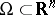, i.e.,(a1)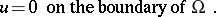(a2)

(Cf. also Dirichlet boundary conditions; Dirichlet eigenvalue.) Here,is the Laplace operator andis an open bounded subset of(). If, the Dirichlet eigenvalues are proportional to the square of the eigenfrequencies of an elastic, homogeneous, vibrating membrane with fixed boundary (cf. also Natural frequencies).

The Rayleigh–Faber–Krahn inequality for the membrane (i.e.,) states that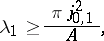(a3)

whereis the first zero of the Bessel function of order zero (cf. also Bessel functions) andis the area of the membrane. Equality is attained in (a3) if and only if the membrane is circular. In other words, among all membranes of given area, the circle has the lowest fundamental frequency. This inequality was conjectured by Lord Rayleigh [a14], based on exact calculations for simple domains and a variational argument for near circular domains. In 1918, R. Courant [a5] proved the weaker result that among all membranes of the same perimeter, the circular one yields the least lowest eigenvalue, i.e.,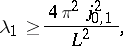(a4)

with equality if and only if the membrane is circular. Rayleigh's conjecture was proven independently by G. Faber [a6] and E. Krahn [a7]. The corresponding isoperimetric inequality in dimension,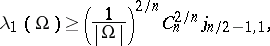(a5)

was proven by Krahn [a8]. In (a5),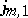is the first positive zero of the Bessel function,is the volume of the domain and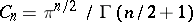is the volume of the-dimensional unit ball. Equality is attained in (a5) if and only ifis a ball.

The proof of the Rayleigh–Faber–Krahn inequality rests upon two facts: a variational characterization for the lowest Dirichlet eigenvalue and the properties of symmetric decreasing rearrangements of functions. The variational characterization of the lowest eigenvalue is given by(a6)

Concerning decreasing rearrangements, letbe a measurable subset of, then the symmetrized domain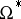is a ball with the same measure as. Ifis a real-valued measurable function defined on a bounded domain, its spherical decreasing rearrangement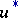is a function defined on the ballcentred at the origin and having the same measure as, such thatdepends only on the distance from the origin, is decreasing away from the origin and is equi-measurable with. See [a13], [a18], [a4] for properties of rearrangements of functions. Since the functionand its spherical decreasing rearrangement are equi-measurable, their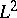-norms are the same. What Faber and Krahn actually proved is that the-norm of the gradient of a function decreases under rearrangements (see [a18] for details, and also [a9] for a different approach to this fact). The fact that the-norm of the gradient of a function decreases under rearrangements, combined with the variational characterization (a6), immediately gives the Rayleigh–Faber–Krahn inequality.

## Isoperimetric inequalities for the lowest eigenvalue.

There are several isoperimetric inequalities for the lowest eigenvalue of boundary value problems, similar to the Rayleigh–Faber–Krahn inequality. The lowest non-trivial Neumann eigenvalue also satisfies an isoperimetric inequality. Letbe the Neumann eigenvalues of the Laplace operator in, i.e.,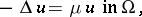(a7)(a8)

If, G. Szegö [a17] proved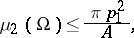(a9)

where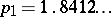, with equality if and only ifis a circle. The corresponding result for dimension,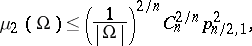(a10)

was proven by H.F. Weinberger [a19], with equality if and only ifis a ball. Here,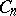is the volume of the unit ball in dimension. In (a9) and (a10),denotes the first positive zero of the derivative of the Bessel function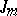. For, Weinberger [a19] also proved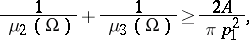(a11)

with equality if and only ifis a circle.

There is also an analogue of the Rayleigh–Faber–Krahn inequality for domains in spaces of constant curvature [a15]. The optimal Rayleigh–Faber–Krahn inequalities for domains in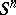was proven by E. Sperner [a16].

In [a14], Lord Rayleigh also conjectured an isoperimetric inequality for the lowest eigenvalue,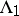, of the clamped plate. The eigenvalue problem for the clamped plate is given bywith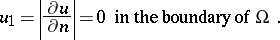Here,is a bounded open subset of. Rayleigh's conjecture for the clamped plate reads(a12)

whereis a ball of the same area as. Rayleigh's conjecture was proven by N. Nadirashvili [a12]. Equality is attained in (a12) if and only ifis a circle. For dimension, the corresponding isoperimetric inequality was proven by M.S. Ashbaugh and R.D. Benguria [a2]. To prove the analogous result for dimensionsand higher is still an open problem (as of 2000, see [a3] however).

Back in the membrane problem, if one goes beyond the lowest eigenvalue, there are several isoperimetric inequalities as well as a number of open problems. The simplest combination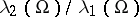satisfies the following inequality [a1]: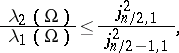(a13)

indimensions, where equality is obtained if and only ifis a ball. Stability results for both the Rayleigh–Faber–Krahn inequality (a3), (a4) and inequality (a13) have been obtained by A.D. Melas [a11] (in simple words, "stability" means that ifis convex and the appropriate left-hand side on either (a3), (a4) or (a13) is not too different from its corresponding isoperimetric value, thenis approximately a ball).